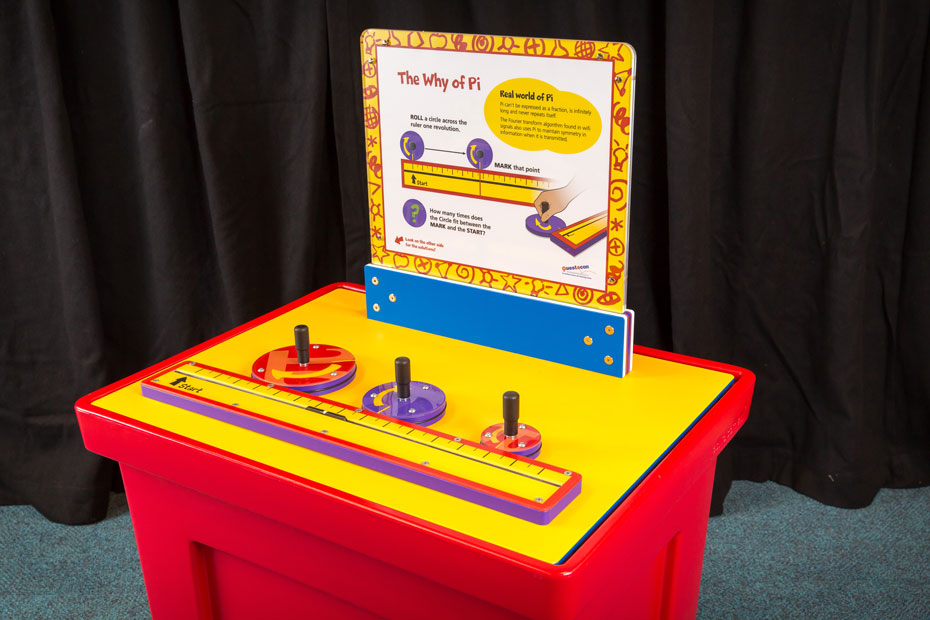# The Why of PiExplore the famous physical and mathematical constant π in this hands-on exhibit.

## How it Works

Rotate a wheel along a line to find the length of the wheel's circumference and discover the origins of π.

## Things to Try or Ask Around the Exhibit

• How many times does the wheel fit along the line?
• Is it a whole number?
• Does the size of the wheel matter?

## Background

π is the ratio between a circle's circumference and its diameter, so the number of times you can fit the wheel inside the line is exactly π.

## Finding the Science in Your World

π is studied and used widely in physics and mathematics, due both to its applications in real systems and interesting properties as a number.